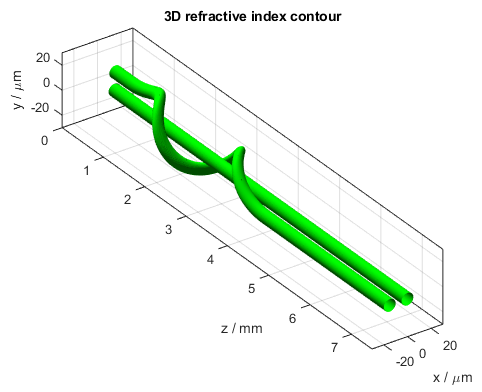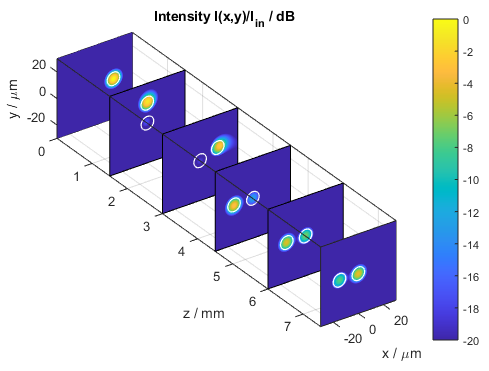﻿ Optical simulation of a spiral waveguide - BeamLab

# Spiral Waveguide

This BeamLab demo shows the behavior of the mode field when a waveguide that is wrapped around a straight waveguide changes to a parallel waveguide.

BeamLab demo: spiral.m

The propagation structure consists of three sections. The first section is a straight single-core waveguide located at x = 15 µm. The second section consists of two waveguides of which the first one is a straight waveguide located at the center of the simulation region, i.e., x = y = 0 µm, and the second one is wrapped around the first one at a center-to-center distance of 15 µm. The third and last section contains two parallel waveguides located at x = -15 µm and x = 0 µm, respectively.3D refractive index contour of the spiral waveguide

The following video shows how the intensity distribution changes along the propagation distance z. The figure shows the two-dimensional intensity distribution I(x,y) normalized to the input intensity and displayed on a logarithmic scale from -20 dB to 0 dB. The white lines indicate the location of the waveguide.

Video of the intensity profile of the beam propagating in the spiral waveguide

The following figure shows a compact three-dimensional view of stacked plots, each portraying the intensity distribution I(x,y) at different distances z.Intensity distribution in the meander waveguide (stacked xy slices)